### Home > CALC > Chapter Ch7 > Lesson 7.3.5 > Problem7-162

7-162.
1.No calculators! Suppose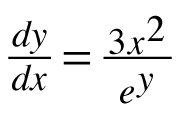. Homework Help ✎

1. Find the particular solution of this differential equation containing the point (0,3).

2. If you have not already done so, solve your equation for y.

3. Find the domain and range of your equation.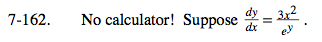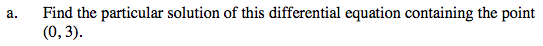Cross multiply in order to separate the variables.

Integrate both sides.

Solve for C by evaluating the given point.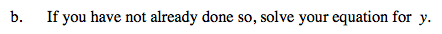y = ln(x3 + e3)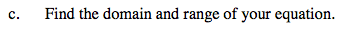The domain is the set of values x can be so that y is still defined.
The range is the set of y values resulting from inputting all members of the domain in the function.

The domain of the parent graph, y = lnx, is x > 0.